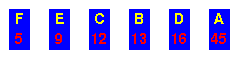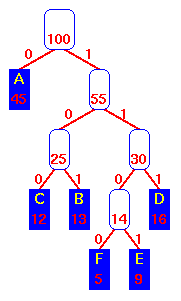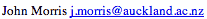Data Structures and Algorithms Operation of the Huffman algorithm

These diagrams show how a Huffman encoding tree is built using a straight-forward greedy algorithm which combines the two smallest-weight trees at every step.Initial data sorted by frequencyCombine the two lowest frequencies, F and E, to form a sub-tree of weight 14. Move it into its correct place.Again combine the two lowest frequencies, C and B, to form a sub-tree of weight 25. Move it into its correct place.Now the sub-tree with weight, 14, and D are combined to make a tree of weight, 30. Move it to its correct place.Now the two lowest weights are held by the "25" and "30" sub-trees, so combine them to make one of weight, 55. Move it after the A.Finally, combine the A and the "55" sub-tree to produce the final tree. The encoding table is: ``` A 0 C 100 B 101 F 1100 E 1101 D 111 ```

©, 1998